# Representations of Exponential & Logarithmic Functions

Instructor: Michael Eckert

Michael has a Bachelor's in Environmental Chemistry and Integrative Science. He has extensive experience in working with college academic support services as an instructor of mathematics, physics, chemistry and biology.

Quantitatively, a logarithmic function has as its inverse an exponential function. Qualitatively, we will show this inverse relationship both algebraically and graphically.

## Algebra, Graphs, and Inverses

To get an understanding of the relationship between a logarithmic and an exponential function, it is first important to make sure that we have a good working concept of an inverse, particularly what a function and its inverse look like as equations and how to convert freely between the two. It is also important to see what this relationship looks like graphically to get an even stronger concept of the inverse. Once these concepts are solidified, we can move on to the inverse relationship (both graphic and algebraic) between a logarithmic function (in this case log base 10) and it respective inverse.

## Review of Inverse Relationships

### Viewing Inverse Relationships Algebraically

Here are some quick examples of functions and their respective inverses. If we are given y = 2x, we find the inverse by simply switching the placement of x and y, which gives us x = 2y. Then we solve for y: y = x / 2. Therefore, the inverse of y = 2x is y = x / 2. Similarly, if we are given y = 5x, the inverse function would be y = x / 5.

How about dealing with a power function (involving an exponent) such as y = x2 ?

We apply the same logic: Switching x for y to give us x = y2.

Solving for y, we have y = √x, a root function.

### Viewing Inverse Relationships Graphically

Graphing in mathematics is a way of visually representing functions in space. Just as an equation can represent and be used to solve real-world problems, so can a function's graphical representation.

Let's look at the relationship between a function and its inverse graphically. First, we will show a graph of a particular function and then, we will add the graph of the inverse of that function. Looking at y = 2x graphically we see: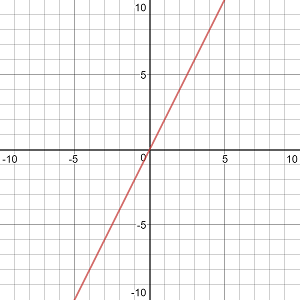Now we add in the inverse y = x / 2 (in blue).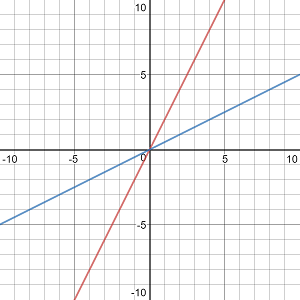The graph of y = x / 2 seems to appear graphically as y = 2x turned backward and upside down. In mathematical terms, their x and y-axis (or their domain and their range) are inverted. Likewise, the same happens with the graph of y = 5x: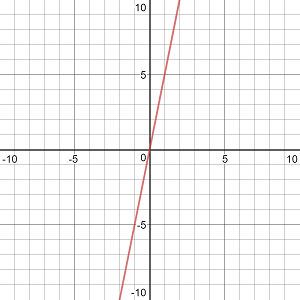Adding in its inverse, y = x / 5 (in blue):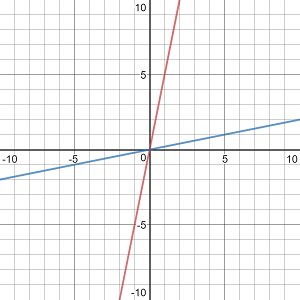Graphically or qualitatively, this inversion of the domain and range is even clearer when we look at a power function (a function with an exponent) and its inverse. Let's start with y = x2

Notice that it is a simple parabola.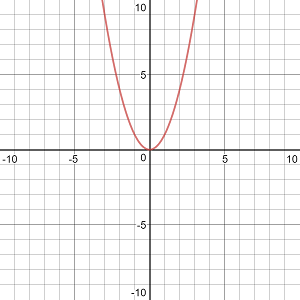Now let's add its inverse y = √x (in blue):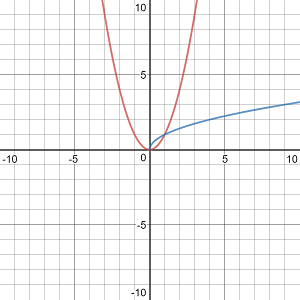Notice that y = √x has no negative x-values, as one cannot have a square root of a negative in the real number system.

## Logarithm and Exponential Functions

Finding the inverse of equations representing lines (both straight and parabolic) seems to be relatively easy. How does the logarithmic function behave when dealing (algebraically and graphically) with its inverse?

To get acquainted with a logarithmic function and its inverse we are going to focus primarily on the general/common logarithmic function with a base of 10 and its inverse.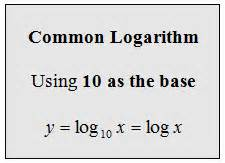Now that we have the common logarithm, how do we find its inverse?

To unlock this lesson you must be a Study.com Member.

### Register to view this lesson

Are you a student or a teacher?